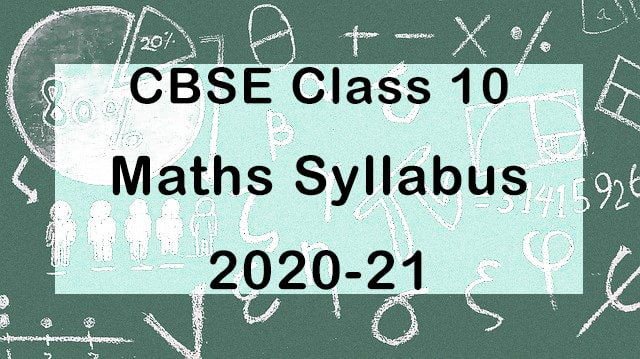## CBSE Class 10 Maths Syllabus 2020-21| Check Latest Exam Pattern

CBSE Class 10 Maths Syllabus 2020-21 has been released officially. Before starting the session, you must need to look at the latest exam pattern to assess yourself effectively. The Maths has been divided into two parts just like previous session into basic and standard. Last year, students who opted for Basic Mathematics are low but CBSE still continued this part.Due to Coronavirus, CBSE has decided to reduce the syllabus by approx 30% overall and this affected Maths syllabus also. There are variety of topics that have been deleted from the syllabus.

 Units Unit Name Marks I Number Systems 06 II Algebra 20 III Coordinate Geometry 06 IV Geometry 15 V Trigonometry 12 V Mensuration 10 VI Probability 11 Total 80

Unit - I - Number Systems

• Real Numbers

Unit - II - Algebra

• Polynomials
• Pair of Linear Equations in two variables
• Arithmetic Progressions

Unit - III - Coordinate Geometry

• Coordinate Geometry

Unit - IV - Geometry

• Triangles
• Circles
• Constructions

Unit - V - Trigonometry

• Introduction to Trigonometry
• Trigonometric Identities
• Heights and Distances

Unit - VI - Mensuration

• Areas related to Circles
• Surfaces, Areas and Volumes

Unit - VII - Statistics & Probability

• Statistics
• Probability

Important Theorems

• If a line is drawn parallel to one side of a triangle to intersect the other two sides in distinct points, the other two sides are divided in the same ratio.
•  The ratio of the areas of two similar triangles is equal to the ratio of the squares of their corresponding sides.
• In a right triangle, the square on the hypotenuse is equal to the sum of the squares on the other two sides.
• In a triangle, if the square on one side is equal to sum of the squares on the other two sides, the angles opposite to the first side is a right angle.
• The tangent at any point of a circle is perpendicular to the radius through the point of contact.
• The lengths of tangents drawn from an external point to a circle are equal.

Topics that have been removed from Class 10 Maths Syllabus 2020-21

• Real Numbers - Euclid’s division lemma.
• Polynomials - Statement and simple problems on division algorithm for polynomials with real coefficients.
• Pair of linear equations in two variables - Cross multiplication method.
• Quadratic equations - Situational problems based on equations reducible to quadratic equations.
• Arithmetic progressions -  Application in solving daily life problems based on sum to n terms
• Coordinate geometry - Area of a triangle.
• Triangles -Proof of the following theorems are deleted
→ The ratio of the areas of two similar triangles is equal to the ratio of the squares of their corresponding sides.
→ In a triangle, if the square on one side is equal to sum of the squares on the other two sides, the angle opposite to the first side is a right angle.
• Circles - No deletion
• Constructions - Construction of a triangle similar to a given triangle.
• Introduction to Trigonometry - motivate the ratios whichever are defined at 0° and 90°.
• Trigonometric identities - Trigonometric ratios of complementary angles.
• Heights and distances - No deletion.
• Areas related to circles - Problems on central angle of 120°
• Surface areas and Volumes - Frustum of a cone.
• Statistics - Step deviation Method for finding the mean, Cumulative Frequency graph
• Probability - No deletion

Prescribed Books:

• NCERT Maths Textbook - Class X
• Laboratory Manual - Mathematics, secondary stage - NCERT Publication
• Mathematics Exemplar problems for class X, NCERT publication